# WebMO Help - Huckel Orbitals

## Extended Huckel Theory

Huckel molecular orbital (HMO) theory is a very simple theory for describing pi-molecular orbitals of planar conjugated carbon systems. Applying the variational method to the Hamiltonian results in the secular equation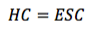which can be solved algebraically for energies En and eigenvectors cn. HMO includes only out-of-plane 2p carbon orbitals where α is the Coulomb energy of a carbon 2p orbital, β is the resonance energy between adjacent out-of-plane carbon 2p orbitals, and non-diagonal overlap integrals are assumed to be zero for mathematical simplicity.

Extended Huckel theory (EHT) extends the Huckel method to atoms beyond carbon, to 3-dimensional molecules, and describes sigma, pi, and delta bonding. EHT solves the same secular equation but uses a minimal basis of atomic orbitals where the Coulomb energy Hii of each orbital is the negative ionization energy of an electron in that orbital, resonance integrals Hij between orbitals are approximated, and overlap integrals Sij are explicitly calculated.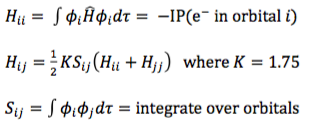EHT contains the essential elements of molecular orbital theory at a minimalistic level. Although not quantitatively accurate, EHT typically offers a qualitatively useful description of the electronic structure in molecules with very low computational demand.

WebMO includes Extended Huckel Theory for the valence electrons, so that low-level quantum chemistry calculations can be performed by a web browser without having to use an external computational chemistry engine. The goal of WebMO's EHT implementation is computational efficiency, not accuracy.

## Calculating Huckel Orbitals

WebMO offers the ability to calculate and display extended Huckel orbitals without invoking a computational engine. Click the Huckel Orbitals icon (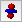) in the toolbar, or choose the Calculate: Huckel: Molecular Orbitals menu item. WebMO will calculate and list the orbitals.

WebMO can also calculate and display electron density surfaces, electrostatic potential maps, and frontier density maps based on the EHT calculation by clicking on the Properties category on the left and selecting the desired surface.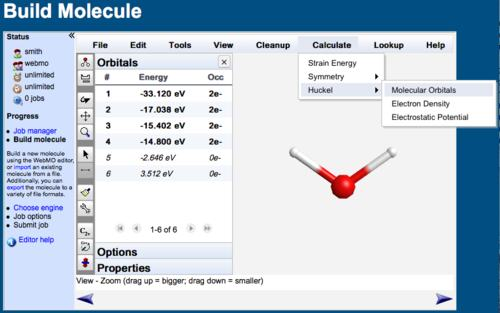Calculating Extended Huckel Orbitals

## Displaying Huckel Orbitals

The viewer for extended Huckel orbitals is the same as the viewer for displaying molecular orbitals calculated by external computational engines.

Click the desired orbital or surface from the list on the left, and it will be displayed in the window. The orbital can be rotated (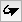), translated (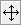), or zoomed (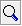) using the toolbar and dragging with the mouse. Isosurface values and transparency can be set with File:Preferences... or the Preferences icon (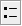). The electrostatic potential color map can be specified with the Options category on the left.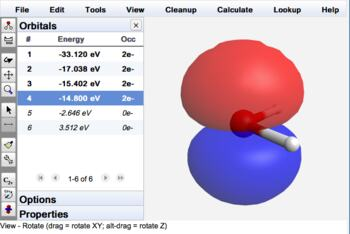Water MO 4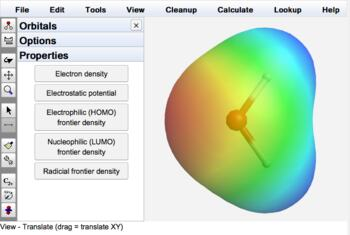Water Electrostatic Potential

Combining mechanics cleanup with a Huckel orbital calculation is a quick way to optimize geometry and view molecular orbitals without using a computational engine. The free WebMO app for smartphones and tablets uses this approach to locally calculate and display molecular orbitals.